# Applications of Integrals Test Paper

1.

Find the area or the region bounded by the curves y = x3 and y = 4x. (5 points)

 0 2 4 8

2.

Find the area of the region bounded by the graphs of y = -x2 + 3x + 4 and y = 4. (5 points)

 2.7 4.5 28.5 16.5

3.

Find the area of the region bounded by the curves y = x2 – 1 and y = sin(x). Give your answer correct to 2 decimal places. (5 points)

4.

The region in the first quadrant bounded by the x-axis, the line x = π, and the curve y = sin(sin(x)) is rotated about the x-axis. What is the volume of the generated solid? (5 points)

 1.219 3.83 1.786 5.612

5.

Which of the following integrals will find the volume of the solid that is formed when the region bounded by the graphs of y = ex, x = 1, and y = 1 is revolved around the line y = -2. (5 points)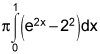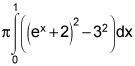6.

The base of a solid in the xy-plane is the circle x2 + y2 = 16. Cross sections of the solid perpendicular to the y-axis are semicircles. What is the volume, in cubic units, of the solid? (5 points)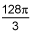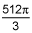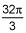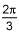7.

Find the average value of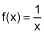over the interval [1, 3]. (5 points)

 0.67 1.1 0.55 0.33

8.

Determine if the Mean Value Theorem for Integrals applies to the function f(x) = 3 – x2 on the interval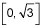. If so, find the x-coordinates of the point(s) guaranteed by the theorem. (5 points)

 No, the Mean Value Theorem for Integrals does not apply Yes, x = 1 Yes, x = 2 Yes,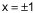9.

For an object whose velocity in ft/sec is given by v(t) = -t2 + 2, what is its displacement, in feet, on the interval t = 0 to t = 2 secs? (5 points)

 1.333 2.438 2.667 -4

10.

For an object whose velocity in ft/sec is given by v(t) = -3t2 + 6, what is its distance travelled, in feet, on the interval t = 0 to t = 2 secs? (5 points)

 4 7.314 3.657 -6

1.

Find the area of the region bounded by the curves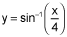, y = 0, and x = 4 obtained by integrating with respect to y. Your work must include the definite integral and the antiderivative. (10 points)

2.

Set up, but do not evaluate, the integral which gives the volume when the region bounded by the curves y = Ln(x), y = 1, and x = 1 is revolved around the line y = -3. (10 points)

3.

The base of a solid in the xy-plane is the first-quadrant region bounded y = x and y = x2. Cross sections of the solid perpendicular to the x-axis are semicircles. What is the volume, in cubic units, of the solid? (10 points)

4.

Determine if the Mean Value Theorem for Integrals applies to the function f(x) = x3 – 4x on the interval [-1, 1]. If so, find the x-coordinates of the point(s) guaranteed to exist by the theorem. (10 points)

5.

An object has a constant acceleration of 40 ft/sec2, an initial velocity of -20 ft/sec, and an initial position of 10 ft. Find the position function, s(t), describing the motion of the object. (10 points)